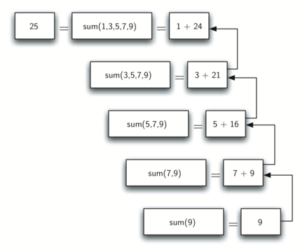TORONTO KIDS COMPUTER CLUB | Aurora Wednesday 18:30 Python Practice 21.12.08.
20141

# Aurora Wednesday 18:30 Python Practice 21.12.08.

## 11 Dec Aurora Wednesday 18:30 Python Practice 21.12.08.

Question:

Use the recursion we have learn in the class, please try to create a function to recursively calculate the total of 1 + 2 + …. (n-2) + (n-1) + n

```def total(n):
# please write you program below

Class Notes:

All recursive algorithms must obey three important laws:

1. A recursive algorithm must have a base case.
2. A recursive algorithm must change its state and move toward the base case.
3. A recursive algorithm must call itself, recursively.

Sample program:

```def listsum(numList):
if len(numList) == 1:
return numList
else:
return numList + listsum(numList[1:])

print(listsum([1,3,5,7,9]))```